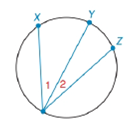Chapter 6.4, Problem 22EElementary Geometry For College St...

7th Edition
Alexander + 2 others
ISBN: 9781337614085

Solutions

Chapter
SectionElementary Geometry For College St...

7th Edition
Alexander + 2 others
ISBN: 9781337614085
Textbook Problem

a) If m X Y ⌢ > m Y Z ⌢ , write an inequality that compares the measures of inscribed angles 1 and 2 .b) If m ∠ 1 < m ∠ 2 , write and inequality that compares the measures of X Y ⌢ and Y Z ⌢ .To determine

(a)

To write:

An inequality that compares the measures of inscribed angles 1 and 2.

Explanation

Given:

mXY>mYZ and the figure given below

Theorem Used:

According to the following theorem

In a circle (or in congruent circles) containing two unequal arcs, the larger arc corresponds to the larger central angle.

Calculation:

Using following theorem

Since the arcs are unequal i.e. mXY>mYZ and from the given figure

To determine

(b)

To write:

An inequality that compares the measures of XY and YZ.

Still sussing out bartleby?

Check out a sample textbook solution.

See a sample solution

The Solution to Your Study Problems

Bartleby provides explanations to thousands of textbook problems written by our experts, many with advanced degrees!

Get Started

Find more solutions based on key concepts